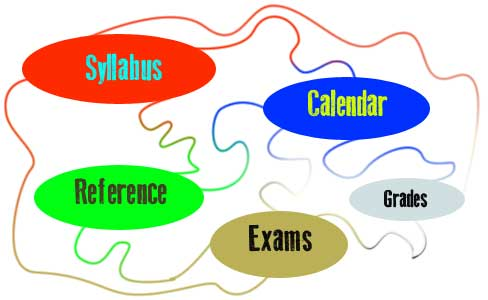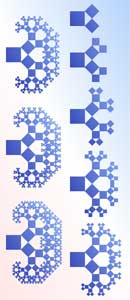Discrete Structures - Spring, 2012# Syllabus—Spring 2012

Instructor: Geoff Hagopian,
email: ghagopian@collegeofthedesert.edu
phone: 760-776-7223

Catalog Description: This course is an introduction to discrete mathematics and its applications. Topics to be covered include logic and sets, relations and functions, combinatorics, probabilities, graph and tree theory, recurrence relations, Boolean algebra, Proofs, algorithms, and finite-state machines. Applications to computer studies and other related areas will be presented. discrete mathematical systems including methods of proof that shape the foundations of computer science. Includes propositional logic, set and number theory, Boolean Algebra, deductive and inductive proof, functions and relations, combinatorics, discrete probability, graph theory and network models, and efficiency of algorithms.

Learning Outcomes:
 1. Evaluate the truth and falsity of mathematical statements employing deductive and inductive proof techniques. 2. Analyze the relationships among counting techniques (combinatorics), discrete probability, sets, Boolean algebra, propositional logic, and the construction of digital circuits. 3. Evaluate graphs, trees, and networks in terms of efficiency, redundancy, and similiarity. 4. Evaluate and prove the efficiency of computer algorithms.

Course Objectives: Upon completion of this course, students will be able to:
1. Apply the principles of mathematical induction, direct and indirect deductive methods of proof to explore integers, rationals, and reals, and their relationships (number theory).
2. Provide recursive, iterative and explicit solutions to classic discrete mathematical problems.
3. Illustrate functional similarities of set theory, discrete probability, propositional logic, Boolean algebra, and digital circuits.
4. Apply graph theory and principles of combinatorial analysis to network models.
5. Create and manipulate trees and specifically spanning trees to find their minimized forms.
6. Create and search Eulerian and Hamiltonian graphs.
7. Identify and solve discrete probability and combinatorial problems.
8. Identify and solve recurrence relations including equivalence relations and partial orderings.
9. Compare the efficiency of common sorting and searching algorithms in terms of
big-O, big-Omega, and big-Theta notation.
10. Convert mathematical algorithms into computer programs.

Textbook: Essentials of Discrete Mathematics: by David J. Hunter ISBN-13: 9781449604424.

Assignments:

· Homework

Weekly assigned reading and exercises are designed to engage your open mind and develop your understanding of course topics.  The Learner, who wishes to try the questions fairly, would do well to follow this guide:
(1) Begin at the beginning, and do not allow yourself to dive into the middle of a difficult topic, only to abandon it because it's "too hard," and thus losing the chance of adding a very large item to your stock of mental delights.
(2) Don't satisfy yourself with a shallow, superficial acquaintance with a topic - instead, seek a thorough understanding and make sure you have correctly worked the examples which have been set.
(3) If you come by some reading you don't understand, read it again. If you don't understand it after a second reading, read it a third time. If you still don't understand it, very likely your brain is getting a little tired. Put the book away for a while and then come back to it when you are rested. You may find it quite easy to understand then.
(4) If possible, find some genial friend who will read the book along with you, and will talk over the difficulties with you. Talking is a wonderful smoother-over of difficulties.

· In-class Activities: These may include on-line or pencil/paper quizzes in various formats such as multiple choice, fill-in-the-blank, short essay, and so on, designed to help you get acquainted with the vocabulary and theories of discrete structures. During lab time we will play games, goof around, design and implement algorithms and experiment with software.

·  Exams: There will be midterm in-class exams and a final in-class exam.

Grading: Homework and Quizzes: 65%; Exams: 35% (20% midterms, 15% final)